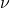# An Auxiliary Variable Trick for MCMC

Robert Nishihara1 Comment

I recently uploaded the paper “Parallel MCMC with Generalized Elliptical Slice Sampling” to the arXiv. I’d like to highlight one trick that we used, but first I’ll give some background. Markov chain Monte Carlo (MCMC) is a class of algorithms for generating samples from a specified probability distribution(in the continuous setting, the distribution is generally specified by its density function). Elliptical slice sampling is an MCMC algorithm that can be used to sample distributions of the form

(1)whereis a multivariate Gaussian prior with meanand covariance matrix, andis a likelihood function. Suppose we want to generalize this algorithm to sample from arbitrary continuous probability distributions. We could simply factor the distributionas

(2)for any Gaussian. In this setting,won’t be a prior, andwon’t be a likelihood function, but this is enough to apply elliptical slice sampling.

Will this work well? Probably not. Elliptical slice sampling works because it makes use of the structure induced by the Gaussian prior. For an arbitrarily chosen Gaussian, this won’t be the case. On the other hand, if we choose a Gaussian that closely approximates, then elliptical slice sampling will probably work well.

This reasoning motivates us to build a Gaussian approximation to. The better our approximation, the better we can expect the sampling algorithm to work. Gaussians all have light tails (the density function diminishes exponentially  as). So, in order to give ourselves more flexibility, we broaden our class of approximations to the class of multivariatedistributions. A multivariatedistribution with parameters,, and(referred to as the degrees of freedom, mean, and covariance parameters respectively) can be written (somewhat suggestively) as

(3)whereis the density function of an inverse-gamma distribution. Now, using a multivariateapproximation, we can write our original density function as

(4)Packaging upas a likelihood function, we are left with the task of generating samples from the probability distribution given by

(5)How can this be done? Here’s the trick. Define a joint distribution

(6)so we have

(7)This tells us that the marginal distribution ofinis our target distribution. Therefore, to generate samples from, it suffices to generate samples fromand then to disregard thecoordinate. In order to generate samples from, we can alternately sample the conditional distributions

(8)and

(9)The first conditional can be sampled with elliptical slice sampling. The second conditional is still an inverse gamma distribution (because the inverse gamma turns out to be a conjugate prior), and so it can be sampled exactly. This approach allows us to generalize elliptical slice sampling to arbitrary continuous distributions.

## One Comment on “An Auxiliary Variable Trick for MCMC”

1.Looks great. Are there certain kinds of continuous distributions that you think will work better than others with this method? What ultimately determines the performance you can expect from this scheme vs some other ‘advanced’ MCMC method (HMC, etc.)?